# 第八章：滤波和卷积¶

## 8.1 平滑¶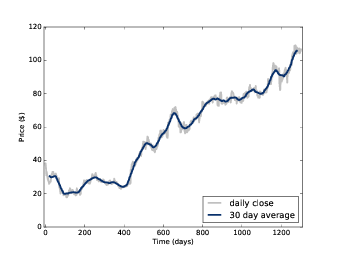signal = thinkdsp.SquareSignal(freq=440)
wave = signal.make_wave(duration=1, framerate=44100)
segment = wave.segment(duration=0.01)


wave 是1s的波形， segment 是一个较短的波形（0.01s），我会用它来作图。

window = np.ones(11)
window /= sum(window)


ys = segment.ys
N = len(ys)
sum(prod)


padded 将窗函数尾部添加0来保证其长度与 segment.ys 一致。这种补0的方法，称为 padding

prod 是波形数据与窗函数的乘积， 他们的和其实就是窗口内前11个元素的平均值。 我们需要把窗口滚动到下一个位置。这个例子中，前11个元素都是-1，因此平均值也是-1。

rolled = np.roll(rolled, 1)
prod = rolled * ys
sum(prod)


def smooth(ys, window):
N = len(ys)
smoothed = np.zeros(N)

for i in range(N):
smoothed[i] = sum(rolled * ys)
rolled = np.roll(rolled, 1)
return smoothed


smooth 就是用来保存结果的数组， padded 就是补0后的窗函数， rolledpadded 的一份拷贝，它在每次循环结束后都会右移一个元素。在循环内，我们将 ysrolled 相乘并求和， 然后依次放入 smooth 数组中。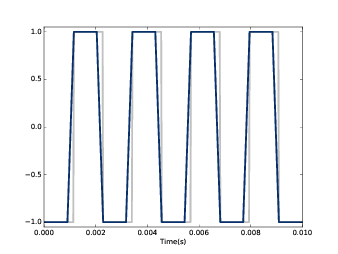## 8.2 卷积¶

convolved = np.convolve(ys, window, mode='valid')
smooth2 = thinkdsp.Wave(convolved, framerate=wave.framerate)


np.convolve 计算了波形和窗函数的卷积。参数 valid 表示只计算窗函数和波形 完全重叠没有交叉的部分，因此它从窗函数与波形数据左对齐开始，直到窗口移动到与波形右对齐。 计算结果与 图8.2 是完全相同的。

$(f \star g)[n] = \sum\limits_{m = 0}^{N - 1} {f[m]g[n + m]}$

$(f * g)[n] = \sum\limits_{m = 0}^{N - 1} {f[m]g[n - m]}$

$$*$$ 是卷积算符，这个式子与之前那个的区别在于 gm 是负的， 也就是 g 的值是反过来的，计算的时候也应该反过来。由于上一个例子中， 窗函数是对称的，因此它们的结果才会一样。如果使用其他的窗函数，就必须要注意这一点。

• 这个定义在很多场合下都是很自然而然的得出来的，尤其是在分析信号处理系统的时候， 我们会在第十章的时候学习。
• 这个定义是卷积定理的基础，我们马上就会学习。

## 8.3 频域¶

spectrum = wave.make_spectrum()
spectrum.plot(color=GRAY)


convolved = np.convolve(wave.ys, window, mode='same')
smooth = thinkdsp.Wave(convolved, framerate=wave.framerate)
spectrum2 = smooth.make_spectrum()
spectrum2.plot()


same 模式表示计算结果需要和输入的长度一致。虽然，这样会产生一些不太好的值， 不过不会应该我们之后的分析。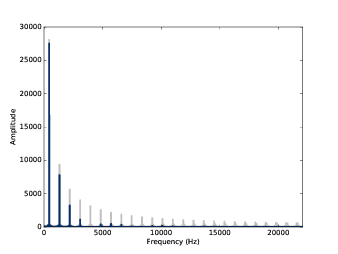amps = spectrum.amps
amps2 = spectrum2.amps
ratio = amps2 / amps
ratio[amps<560] = 0
thinkplot.plot(ratio)


ratio 就是平滑前和平滑后的幅值比例。 当 amps 很小的时候，这个比例会很大并且没什么意义， 因此我们简单的把它设置为了0 。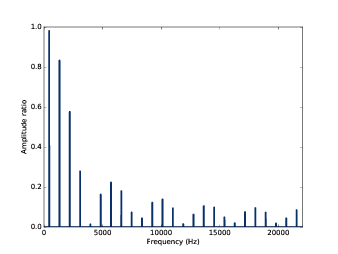## 8.4 卷积定理¶

$DFT(f * g) = DFT(f) \cdot DFT(g)$

• 时域的卷积相当于频域的乘积

padded = zero_pad(window, N)
thinkplot.plot(abs(dft_window))


padded 是补0后的窗函数， dft_window 是窗函数的DFT。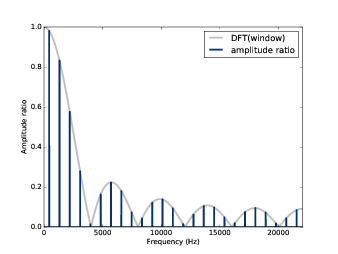$|DFT(f*g)|/|DFT(f)| = |DFT(g)|$

## 8.5 高斯滤波器¶

gaussian = scipy.signal.gaussian(M=11, std=2)
gaussian /= sum(gaussian)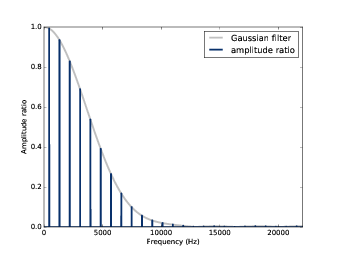## 8.6 高效的卷积¶

FFT的一个重要的运用是，它与卷积定理结合起来可以提供一种高效的计算卷积，互相关和自相关函数的方法。

$DFT(f * g) = DFT(f) \cdot DFT(g)$

$f*g = IDFT(DFT(f) \cdot DFT(g))$

IDFT 指的是逆DFT变换。我们之前使用的卷积计算方式，时间复杂度是 $$O({n^2})$$ ； 而使用这种方式，时间复杂度仅为 $$O(n\log n)$$

import pandas as pd

names = ['date', 'open', 'high', 'low', 'close', 'volume']
ys = df.close.values[::-1]


window = scipy.signal.gaussian(M=30, std=6)
window /= window.sum()
smoothed = np.convolve(ys, window, mode='valid')


from np.fft import fft, ifft

def fft_convolve(signal, window):
fft_signal = fft(signal)
fft_window = fft(window)
return ifft(fft_signal * fft_window)


padded = zero_pad(window, N)


smooth2 的开头包含 M-1 个无效的数据， M 是窗长度，我们可以像下面这样来去掉这些数据:

M = len(window)
smoothed2 = smoothed2[M-1:]


## 8.7 高效的自相关¶

8.2 卷积 中我提到了互相关的定义，它和卷积之间仅有一个符号的差别。

corrs = np.correlate(close, close, mode='same')def fft_autocorr(signal):
N = len(signal)
window = np.flipud(signal)

corrs = fft_convolve(signal, window)
corrs = np.roll(corrs, N//2+1)[:N]
return corrs


fft_convolve 的结果长度是2N，其中前 N/2 个元素和后 N/2 个元素是有效的，其余都是由于补0 导致的无效数据，于是我们将它右移并取出了前 N 个元素，也就对应了 lag-N/2N/2-1 的值。 结果如 图8.8 ，两种计算方法得到的结果是相同的。